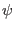Next: Superposition and Eigenstates Up: The Quantum Computer Previous: The Quantum Bit   Contents

## State Vectors and Dirac Notation

We wish to know exactly how the behavior of the spin-1/2 particle, our qubit, differs from a that of a classical bit. A classical bit can store either a 1 or a 0, and when measured the value observed will always be the value stored. Quantum physics states that when we measure the spin-1/2 particles state we will determine that it is in the +1/2 state, or the spin -1/2 state. In this manner our qubit is not different from a classical bit, for it can be measured to be in the +1/2, or 1 state, or the -1/2, or 0 state. The differences between the qubit and the bit come from what sort of information a qubit can store when it is not being measured.

According to quantum physics we may describe that state of this spin-1/2 particle by a state vector in a Hilbert Space. A Hilbert Space is a complex linear vector space.

A complex vector space is one in which the lengths of the vectors within the space are described with complex numbers. A linear vector space is one that you may add and multiply vectors that lie in the space and the resulting vector will still lie within that space. (Williams, Clearwater)

The Hilbert Space for a single qubit will have two perpendicular axes, one corresponding to the spin-1/2 particle being in the +1/2 state, and the other to the particle being in the -1/2 state. These states which the vector can be measured to be are referred to as eigenstates. The vector which exists somewhere in this space which represents the state of the spin-1/2 particle is called the state vector. The projection of the state vector onto one of the axes shows the contribution of that axes' eigenstate to the whole state. This means that in general, the state of the spin-1/2 particle can be any combination of the base states. In this manner a qubit it totally unlike a bit, a bit can exist in only the 0 or 1 state, but the qubit can exist, in principle, in any combination of the 0 and 1 state, and is only constrained to be in the 0 or 1 state when we measured.

Now we introduce the standard notation for state vectors in Quantum physics. The state vector is written the following way is called a ket vector |>. Whereis a list of numbers which contain information about the projection of the state vector onto its base states. The term ket and this notation come from the physicist Paul Dirac who wanted a concise shorthand way of writing formulas that occur in Quantum physics. These formulas frequently took the form of the product of a row vector with a column vector. Thus he referred to row vectors as bra vectors represented as < y|. The product of a bra and a ket vector would be written < y| x >, and would be referred to as a bracket. (Williams, Clearwater)Next: Superposition and Eigenstates Up: The Quantum Computer Previous: The Quantum Bit   Contents
Matthew Hayward - Quantum Computing, Shor's Algorithm, and Parallelism GitHub Repository